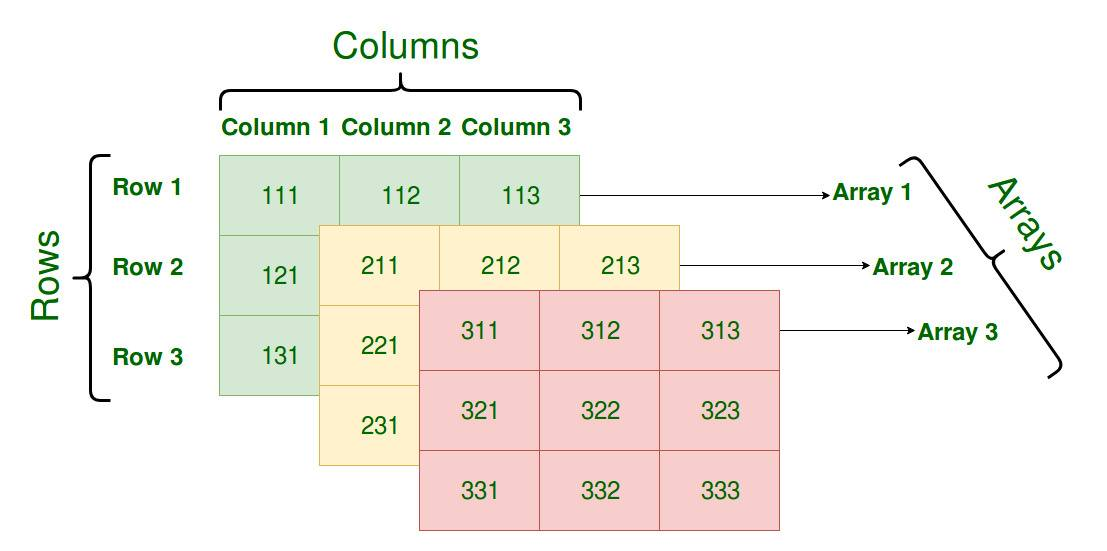设为首页 加入收藏
TOP
Go语言数组和切片的原理(一)
2019-03-26 16:13:29 】 浏览:151
 Tags：语言 切片 原理

数组``````int
interface{}``````

Go 语言中数组的大小在初始化之后就无法改变，数组存储元素的类型相同，但是大小不同的数组类型在 Go 语言看来也是完全不同的，只有两个条件都相同才是同一个类型。

``````func NewArray(elem *Type, bound int64) *Type {
if bound < 0 {
Fatalf("NewArray: invalid bound %v", bound)
}
t := New(TARRAY)
t.Extra = &amp;Array{Elem: elem, Bound: bound}
t.SetNotInHeap(elem.NotInHeap())
return t
}``````

创建

Go 语言中的数组有两种不同的创建方式，一种是我们显式指定数组的大小，另一种是编译器通过源代码自行推断数组的大小：

``````arr1 := int{1, 2, 3}
arr2 := [...]int{1, 2, 3}``````

上限推导

``````func typecheckcomplit(n *Node) (res *Node) {
// ...

switch t.Etype {
case TARRAY, TSLICE:
var length, i int64
nl := n.List.Slice()
for i2, l := range nl {
i++
if i > length {
length = i
}
}

if t.IsDDDArray() {
t.SetNumElem(length)
}
}
}``````

语句转换

``````func anylit(n *Node, var_ *Node, init *Nodes) {
t := n.Type
switch n.Op {
case OSTRUCTLIT, OARRAYLIT:
if n.List.Len() > 4 {
vstat := staticname(t)

fixedlit(inNonInitFunction, initKindStatic, n, vstat, init)

a := nod(OAS, var_, vstat)
a = typecheck(a, ctxStmt)
a = walkexpr(a, init)
init.Append(a)
break
}

fixedlit(inInitFunction, initKindLocalCode, n, var_, init)
// ...
}
}``````
1. 当元素数量小于或者等于 4 个时，会直接将数组中的元素放置在栈上；
2. 当元素数量大于 4 个时，会将数组中的元素放置到静态区并在运行时取出；

``````func fixedlit(ctxt initContext, kind initKind, n *Node, var_ *Node, init *Nodes) {
var splitnode func(*Node) (a *Node, value *Node)
// ...

for _, r := range n.List.Slice() {
a, value := splitnode(r)

a = nod(OAS, a, value)
a = typecheck(a, ctxStmt)
switch kind {
case initKindStatic:
genAsStatic(a)
case initKindLocalCode:
a = orderStmtInPlace(a, map[string][]*Node{})
a = walkstmt(a)
init.Append(a)
default:
}
}
}``````

``````var arr int
arr = 1
arr = 2
arr = 3``````

``````func fixedlit(ctxt initContext, kind initKind, n *Node, var_ *Node, init *Nodes) {
var splitnode func(*Node) (a *Node, value *Node)
// ...

for _, r := range n.List.Slice() {
a, value := splitnode(r)

setlineno(value)
a = nod(OAS, a, value)
a = typecheck(a, ctxStmt)
switch kin

编程开发网 ``````

】【打印繁体】【投稿】【收藏】 【推荐】【举报】【评论】 【关闭】 【返回顶部array(4) { ["type"]=> int(8) ["message"]=> string(24) "Undefined variable: jobs" ["file"]=> string(32) "/mnt/wp/cppentry/do/bencandy.php" ["line"]=> int(217) }